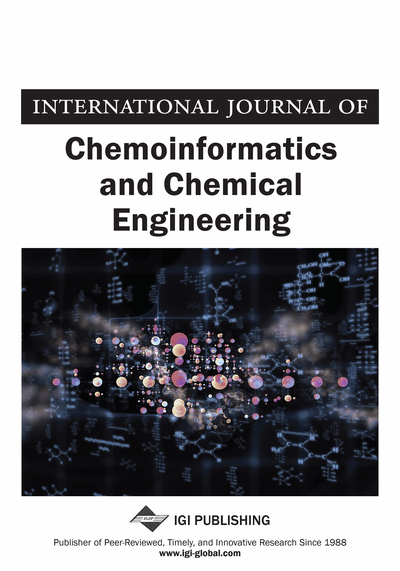# An Efficient Algorithm for Automating Classification of Chemical Reactions into Classes in Ugi’s Reaction Scheme

Sanjay Ram (Bengal Engineering and Science University, Shibpur, India) and Somnath Pal (Bengal Engineering and Science University, Shibpur, India)
DOI: 10.4018/ijcce.2012070101
Available
\$37.50
No Current Special Offers

## Abstract

There are two approaches for classification of chemical reactions: Model-Driven and Data-Driven. In this paper, the authors develop an efficient algorithm based on a model-driven approach developed by Ugi and co-workers for classification of chemical reactions. The authors’ algorithm takes reaction matrix of a chemical reaction as input and generates its appropriate class as output. Reaction matrices being symmetric, matrix implementation of Ugi’s scheme using upper/lower tri-angular matrix is of O(n2) in terms of space complexity. Time complexity of similar matrix implementation is O(n4), both in worst case as well as in average case. The proposed algorithm uses two fixed size look-up tables in a novel way and requires constant space complexity. Time complexity both in worst and average cases of the algorithm is linear.
Article Preview
Top

## Background

Among several representations of chemical structures graph theoretic representation (Ivanciuc, 2010; Kier & Hall, 1999; Trinajstic, 1992) is most suitable for obtaining information using model-driven approach. In the following subsections we have discussed such representation and detailed bond-electron matrix and reaction matrix used in this paper under graph theoretic representation.

### Graph Theoretic Representation of Molecules and Reactions

Chemical structures are usually stored in a computer as molecular graphs (Beck et al., 1969). A graph is an abstract structure that contains nodes connected by edges. One example of graph that corresponds to chemical structure of ethanal is shown in Figure 1. In such molecular graphs the nodes correspond to the atoms and the edges to the bonds. Such graphs can also be represented as matrices. Their major advantage is that the calculation of paths and cycles can be performed easily by well-known matrix operations. The matrix of a structure with n atoms consists of an array of n × n entries. A molecule with its different atoms and bond types can be represented in matrix form in different ways depending on what kind of entries are chosen for the atoms and bonds. Thus, a variety of matrix representations for chemical compounds are in use (Gasteiger & Engel, 2003): adjacency (Ivanciuc, 2000; Markovic et al., 2001), distance (Ivanciuc & Ivanciuc, 1999; Mihalic et al., 1992), incidence, bond, and bond-electron matrices. Among these representations bond-electron matrix representation provides all information given by bond matrix and additionally number of free electrons of an atom. In this work we have used BE-matrix, the representation of which is explained in the following.

## Complete Article List

Search this Journal:
Reset
Open Access Articles
Volume 8: 2 Issues (2019)
Volume 7: 2 Issues (2018)
Volume 6: 2 Issues (2017)
Volume 5: 2 Issues (2016)
Volume 4: 2 Issues (2015)
Volume 3: 2 Issues (2013)
Volume 2: 2 Issues (2012)
Volume 1: 2 Issues (2011)
View Complete Journal Contents Listing